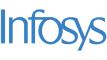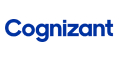New update is available. Click here to update.
Topics

# Sliding Maximum

Moderate0/80
Average time to solve is 25m+14 more companies

## Problem statement

You are given an array 'ARR' of integers of length 'N' and a positive integer 'K'. You need to find the maximum elements for each and every contiguous subarray of size K of the array.

For example
``````'ARR' =  [3, 4, -1, 1, 5] and 'K' = 3
Output =  [4, 4, 5]

Since the maximum element of the first subarray of length three ([3, 4, -1]) is 4, the maximum element of the second subarray of length three ([4, -1, 1]) is also 4 and the maximum element of the last subarray of length three ([-1, 1, 5]) is 5, so you need to return [4, 4, 5].
``````
Detailed explanation ( Input/output format, Notes, Images )
Sample Input 1 :
``````1
5 3
3 2 -6 1 0
``````
Sample Output 1:
``````3 2 1
``````
Explanation for Input 1:
``````The subarray of length 'K'      maximum element of the subarray.
3 2 -6                                    3
2 -6 1                                    2
-6 1 0                                    1

Thus, you need to return "3 2 1".
``````
Sample Input 2 :
``````1
9 3
1 2 3 1 4 5 2 3 6
``````
Sample Output 2:
``````3 3 4 5 5 5 6
``````Console